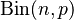# Binomial distribution facts for kids

Kids Encyclopedia Facts

In probability and statistics, the binomial distribution is a probability distribution which models the probabilities of having a certain number of successes among n identical trials (each having p as the probability of success). It is also written as$\operatorname{Bin}(n, p)$. The variables n and p are thus the two parameters of a binomial distribution.

The binomial distribution has discrete values. It counts the number of successes in yes/no-type experiments. Each of these experiment, also called Bernoulli trial, either results in success or failure. Examples of binomial distribution include:

• Tossing a coin 10 times, and counting the number of face-ups. (n=10, p=1/2)
• Rolling a dice 10 times, and counting the number of sixes. (n=10, p=1/6)
• Counting the number of green-eyed people among 500 randomly chosen people (assuming that 5% of all people have green eyes). (n=500, p=0.05)

In order to use the binomial distribution, the following must be true about the problem:

1. The outcomes are mutually exclusive, that is, there are two possible outcomes which cannot occur simultaneously (for example. in flipping a coin, there are two possible outcomes: heads or tails. It is always one or the other, never both or a mix of outcomes).
2. The probability of a success (p) is consistent throughout the problem (for example, a basketball player makes 85% of his free throws. Each time the player attempts a free throw, 85% is assumed to be the likelihood of a made shot).
3. The trials are independent of each other (for example, on the second flip of a coin, the first outcome does not impact the chance of the next toss: the chance of tossing a heads (or tails) is still 50%).

## Related pagesBinomial distribution Facts for Kids. Kiddle Encyclopedia.